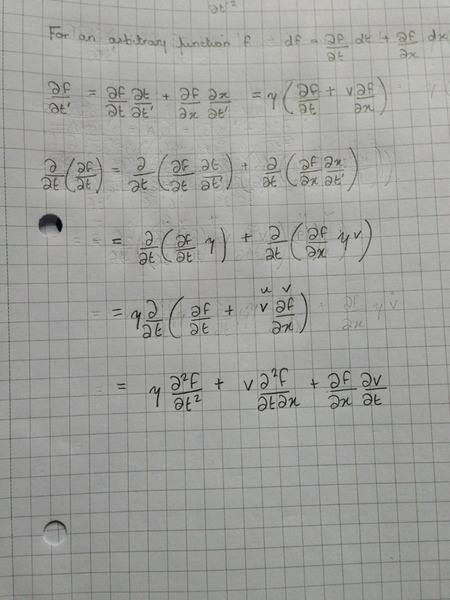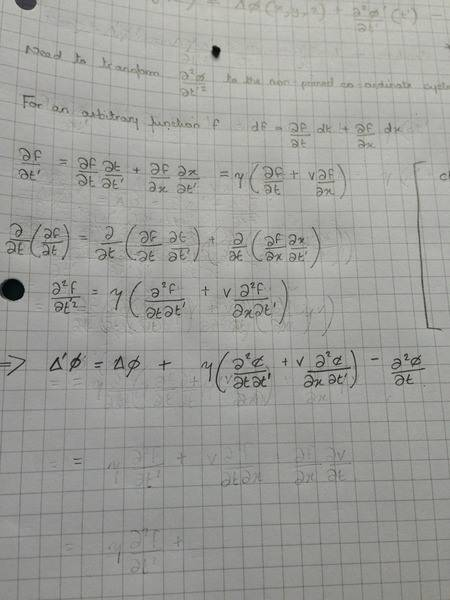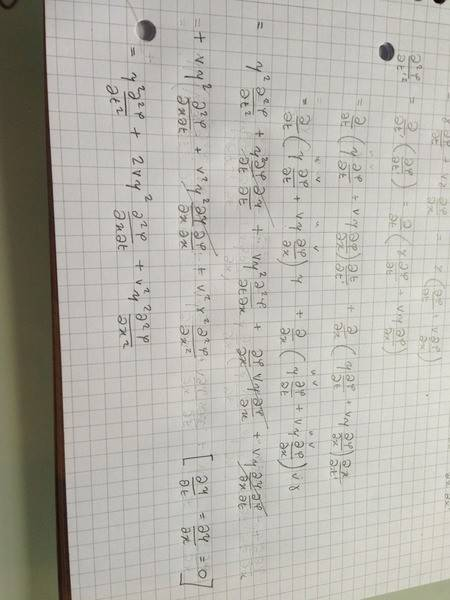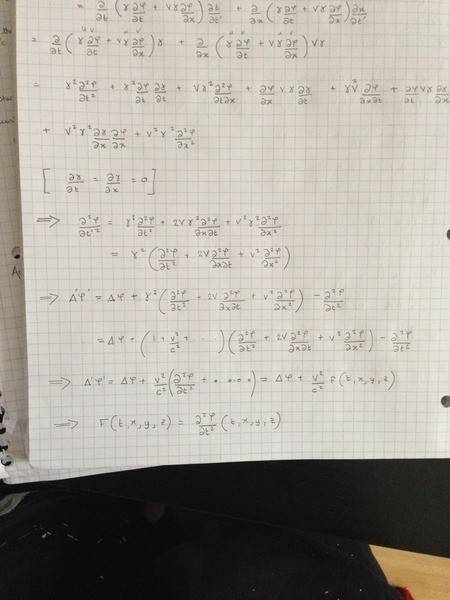# Lorentz Transformations

1.Hey, I am rather stuck on this question which you can see in the attached PDF. Now I began by taylor expanding the Lorentz Gamma factor (γ), up to second order and inserting this into the equation wherever I saw the gamma function, then rearranging. But I can't seem to get a function for F, for me my F comes out to be 1/2

2. The relevant equations can be seen in the PDF

3. I'm new to this, so not sure how to write it all out, however I did a taylor expansion of gamma, then substituted and rearranged and ended up with F as 1/2. I know this is wrong as obviously this would act on the y and z components which it shouldn't, as they are not affected by the transformation.

#### Attachments

• comment05Sept(1).pdf
16.5 KB · Views: 190

Chestermiller
Mentor
Hi smallgirl. Welcome to Physics Forums.

Try making use of equations 1 and 2, and getting $Δ'\phi '-Δ\phi$. This should lead to partials with respect to t and t' only.

Hey,

I don't understand the reasoning in doing this...

Chestermiller
Mentor
Hey,

I don't understand the reasoning in doing this...

$$Δ'\phi'=Δ\phi+\frac{1}{c^2}(\frac{\partial ^2\phi'}{\partial t'^2}-\frac{\partial ^2\phi}{\partial t^2})$$
The only thing you need to do is transform $\frac{\partial ^2\phi'}{\partial t'^2}$ to the non-primed coordinate system.

EDIT: I corrected a minor error in the earlier version of this response.

Last edited:
Would this give me the function F?

Ok so I've been staring at it for a while, not sure how to get ∂2ϕ′/∂t′2 to the non-primed coordinate system

Chestermiller
Mentor
Ok so I've been staring at it for a while, not sure how to get ∂2ϕ′/∂t′2 to the non-primed coordinate system
For an arbitrary function f,
$$df=\frac{\partial f}{\partial t}dt+\frac{\partial f}{\partial x}dx$$
$$\frac{\partial f}{\partial t'}=\frac{\partial f}{\partial t}\frac{\partial t}{\partial t'}+\frac{\partial f}{\partial x}\frac{\partial x}{\partial t'}=γ(\frac{\partial f}{\partial t}+v\frac{\partial f}{\partial x})$$

•1 person
Hey,

Yes I had actually got that, but I didn't think what I was doing was right... I figured I would then need to differentiate again?

Chestermiller
Mentor
Hey,

Yes I had actually got that, but I didn't think what I was doing was right... I figured I would then need to differentiate again?
Yes. You do need to differentiate again. I only presented this result so that I could get you pointed in the right direction. So, differentiate again and show us what you got.

I get zero...

Well ok I get this, but it seems wrong

gamma((d2f/dt'dt)+ V(d2f/dt'dx))

Last edited:, Hey, see the attachment for what I got... Ignore the previous post...

I am really struggling with this :-(... I can't see what I am meant to be doing to get to the answer.. Like I can't see the path

Chestermiller
Mentor
View attachment 61514, Hey, see the attachment for what I got... Ignore the previous post...

I am really struggling with this :-(... I can't see what I am meant to be doing to get to the answer.. Like I can't see the path
You need a little practice doing coordinate transformations. Check this out:

$$\frac{\partial^2 f}{\partial t'^2}=γ(\frac{\partial^2 f}{\partial t\partial t'}+v\frac{\partial^2 f}{\partial x\partial t'})$$

Ahh your kidding me right? I had that originally a you can see from my previous post

Anyways, given that I don't know what f is.. I'm not sure how to proceed

Chestermiller
Mentor
Ahh your kidding me right? I had that originally a you can see from my previous post

Anyways, given that I don't know what f is.. I'm not sure how to proceed
f is just an arbitrary function of the primed and/or the unprimed variables. Just substitute phi for f. Remember that, at a given location in 4D space time, phi prime is the same as phi.

Hey,

Yeah I did that and substituted it in and got thisI'm confused as to where and when I'd do a Taylor expansion? Do I just Taylor expand the Lorentz factor? But that seems a bit wrong....

Chestermiller
Mentor
Hey,

Yeah I did that and substituted it in and got this View attachment 61516

I'm confused as to where and when I'd do a Taylor expansion? Do I just Taylor expand the Lorentz factor? But that seems a bit wrong....
This is not what I got. Check the math. I got:

$$\frac{\partial^2 \phi}{\partial t'^2}=γ^2\left(\frac{\partial ^2 \phi}{\partial t^2}+2v\frac{\partial^2 \phi}{\partial x\partial t}+v^2\frac{\partial^2 \phi}{\partial x^2}\right)$$Hey so I figured the maths out and arrived at what you have..... Not sure what I'm meant to do now... I'm utterly lost....

Hmm maybe taylor expand gamma and then substitute in?

Last edited:
Chestermiller
Mentor
View attachment 61519

Hey so I figured the maths out and arrived at what you have..... Not sure what I'm meant to do now... I'm utterly lost....

OK. You're almost done. Now you substitute this back into the equation relating the Δ's, and combine the two terms containing the second partials with respect to t, by reducing them to a common denominator. It looks like your final result is going to have a linear term in v/c, which apparently the original problem statement omitted. At this point, you can approximate the γ's by 1. You will have 3 terms on the rhs, one with a factor of v/c and two with factors of (v/c)2. In any event, the presence of the linear term in v/c will not change the main conclusion from the problem statement.

Chet

I don't understand what you mean by reducing them to a common denominator..

Chestermiller
Mentor
I don't understand what you mean by reducing them to a common denominator..
$$γ^2-1=\frac{1}{1-(\frac{v}{c})^2}-1=\frac{(\frac{v}{c})^2}{1-(\frac{v}{c})^2}=γ^2(\frac{v}{c})^2$$

Chestermiller
Mentor
As I mentioned earlier, I get a result different from Eqn. 3 in your problem statement. I get
$$Δ'\phi'=Δ\phi+E\left(\frac{v}{c}\right)+F\left(\frac{v}{c}\right)^2+...$$
where
$$E=\frac{2}{c}\frac{\partial^2\phi}{\partial t\partial x}$$
and
$$F=\left(\frac{1}{c^2}\frac{\partial^2\phi}{\partial t^2}+\frac{\partial^2\phi}{\partial x^2}\right)$$

I have confidence in what I did, but no one is perfect. Do you have any idea what answer the book gives? Does it present a solution?

Chet

Hey,

I've yet to go through your solution and see how that works out for me, however this is what I came up with( see attachment)... Not sure how accurate the logic is...either way, I am soooo grateful for your help, so thank you soooo much!

I have no answer to check with...In your solution I have worked through it, but I can't see where you get the ( 1/c^2)from... Right at the begining

Last edited:
Chestermiller
Mentor
In your solution I have worked through it, but I can't see where you get the ( 1/c^2)from... Right at the begining

In special relativity, we either absorb the c within the symbol t (and call t light-years), or we retain the c in the equations (and call t years) but we don't do both. Your problem statement (incorrectly) does both. In the Lorentz Transformation, c is retained, while in the differential equations, c is absorbed. I put the c back into the differential equation so it is consistent with the form of the Lorentz Transformation used in the problem statement. Without the c in the differential equation, if t is regarded as time, the units of time and distance in the differential equation are not consistent.

I looked through your last hand-written development, and there are algebra errors in the last two lines (especially if you correctly include c in these relations).

Chet

Ahh so should I have expanded the whole thing then, and then rearranged?

Chestermiller
Mentor
Ahh so should I have expanded the whole thing then, and then rearranged?
Sure...and don't forget to include those c2's in the denominator.

Chestermiller
Mentor
Ahh so should I have expanded the whole thing then, and then rearranged?
Sure...and don't forget to include those missing c2's.

Hmm.... Arg something tells me I'd be better off starting again from scratch and setting c to 1.... Would the answer be similar to yours but without the c factors?

Chestermiller
Mentor
Hmm.... Arg something tells me I'd be better off starting again from scratch and setting c to 1.... Would the answer be similar to yours but without the c factors?
Yes, but you would have to set the c's equal to 1 in the Lorentz Transformation equations, and set the c's to 1 in the problem statement solution. You would also probably want to change all the v's to betas. Actually, I anticipate a disaster if you try to do this. Actually, all you need to do is put a 1/c2 in front of the time derivative terms in the first equation and everything will then be consistent.

Chet

Last edited:
Hey!

Just want to say, thank you soo much for having patience with me :-). I got the answer you had by adding the 1/c^2 factor and following what you did! Thank you soo much I massively appreciate what you did. Thank you :-)